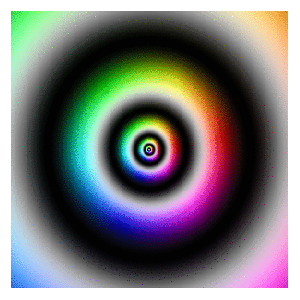# Zooming

You don’t have to be beautiful to turn me on (Kiss, Prince)

I discovered recently how easy is to create GIFs with R using ImageMagick and I feel like a kid with a new toy. To begin this new era of my life as R programmer I have done this:First of all, read this article: it explains very well how to start doing GIFs from scratch. The one I have done is inspired in this previous post where I take a set of complex numbers to transform and color it using HSV technique. In this case I use this next transformation:$f(z)= -Im(z)+(Re(z)+0.5*Im(z))*1i$

Modifying the range of `Real` and `Imaginary` parts of complex numbers I obtain the zooming  effect. The code is very simple. Play with it changing the transformation or the animation options. Send me your creations, I would love to see them:

```library(dplyr)
library(ggplot2)
dir.create("output")
setwd("output")
id=1 # label tO name plots
for (i in seq(from=320, to=20, length.out = 38)){
z=outer(seq(from = -i, to = i, length.out = 300),1i*seq(from = -i, to = i, length.out = 500),'+') %>% c()
z0=z
for (k in 1:100) z <- -Im(z)+(Re(z)+0.5*Im(z))*1i
df=data.frame(x=Re(z0),
y=Im(z0),
h=(Arg(z)<0)*1+Arg(z)/(2*pi), s=(1+sin(2*pi*log(1+Mod(z))))/2, v=(1+cos(2*pi*log(1+Mod(z))))/2) %>% mutate(col=hsv(h,s,v))
ggplot(df, aes(x, y)) +
geom_tile(fill=df\$col)+
scale_x_continuous(expand=c(0,0))+
scale_y_continuous(expand=c(0,0))+
labs(x=NULL, y=NULL)+
theme(legend.position="none",
panel.background = element_rect(fill="white"),
plot.margin=grid::unit(c(1,1,0,0), "mm"),
panel.grid=element_blank(),
axis.ticks=element_blank(),
axis.title=element_blank(),
axis.text=element_blank())